Next: 4.1.3 High Values of Up: 4.1 Unprimed Subbands Previous: 4.1.1 Scaled Energy Dispersion

## 4.1.2 Small Strain Values

The valley splitting was shown to be linear in strain for small shear strain values and to depend strongly on the film thickness . To support these findings we reformulated (4.14) for the sum and the difference ofand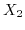. First we introduce the transformation rules forand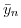as,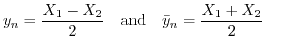(4.17)

or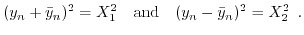(4.18)

We only show the derivation for (4.14a), due to the similarity with (4.14b). Using the above given transformation and rewriting (4.14a) to separateandleads to the following expression.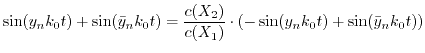(4.19)

Further simplification steps result in:(4.20)

Now we re-expressas function of(Appendix B.) resulting in(4.21)

The derivation of the fraction containing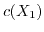and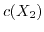can be found in Appendix B..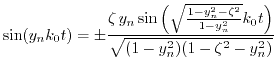(4.22)

For zero stress the ratio on the right hand side of (4.22) is equal to zero, and the standard quantization condition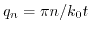is recovered. Due to the plus/minus sign in the right-hand side of (4.22), the equation splits into two non-equivalent branches for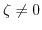and non-parabolic bands. (4.22) is nonlinear and can be solved only numerically. However, for smallthe solution can be thought in the form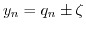, whereis small. Substituting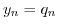into the right-hand side of (4.22) and solving the equation with respect to, we obtain for the valley splitting: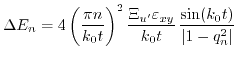(4.23)

In accordance with earlier publications [182,183,184,185], the valley splitting is inversely proportional to the third power of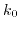and the third power of the film thickness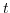. The value of the valley splitting oscillates with film thickness, in accordance with [183,184,185]. In contrast to previous works, the subband splitting is proportional to the gap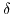at the X-point, and not at the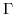-point. Since the parameter, which determines non-parabolicity, depends strongly on shear strain, the application of uniaxial  stress to  ultra-thin Si film generates a valley splitting proportional to strain.Next: 4.1.3 High Values of Up: 4.1 Unprimed Subbands Previous: 4.1.1 Scaled Energy Dispersion

T. Windbacher: Engineering Gate Stacks for Field-Effect Transistors